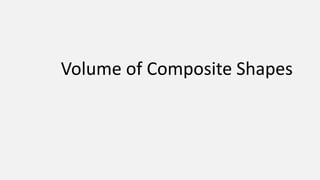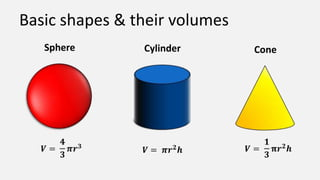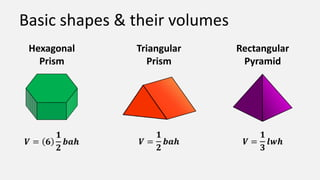Successfully reported this slideshow.

# Mat2793 - Volume of Composite Shapes×

1 of 38
1 of 38

# Mat2793 - Volume of Composite Shapes

## More Related Content

### Mat2793 - Volume of Composite Shapes

1. 1. Volume of Composite Shapes
2. 2. Basic shapes & their volumes Sphere Cylinder Cone
3. 3. Basic shapes & their volumes Hexagonal Triangular Rectangular Prism Prism Pyramid
4. 4. What is a composite shape?
5. 5. Two basic shapes added together This house is a triangular prism on top of a rectangular prism
6. 6. Two basic shapes added together This house is a triangular prism on top of a rectangular prism
7. 7. One shape subtracted from another This hexagonal prism becomes a nut
8. 8. One shape subtracted from another This hexagonal by removing a cylinder prism becomes a from its center nut
9. 9. A shape that is truncated (sliced) This cup
10. 10. A shape that is truncated (sliced) This cup is a large cone with a small cone removed
11. 11. A shape that is truncated (sliced) This cup is a large cone with a small cone removed
12. 12. A shape that is truncated (sliced) This cup is a large cone with a small cone removed
13. 13. A closer look at adding shapes
14. 14. Warm-up: Can you identify the basic shapes in the following examples?
15. 15. Example - Ice cream cone
16. 16. Example - Ice cream cone cone
17. 17. Example - Ice cream cone cone
18. 18. Example - Ice cream cone cone
19. 19. Example - Traffic cone
20. 20. Example - Traffic cone cone rectangular prism
21. 21. Example - Traffic cone cone rectangular prism
22. 22. Example - Traffic cone cone rectangular prism
23. 23. Example – Washington Monument
24. 24. Example – Washington Monument pyramid rectangular prism
25. 25. Example – Washington Monument pyramid rectangular prism
26. 26. Example – Washington Monument pyramid rectangular prism
27. 27. Example - Hopper bin
28. 28. Example - Hopper bin cylinder cones
29. 29. Example - Hopper bin cylinder cones
30. 30. Example - Hopper bin cylinder cones
31. 31. Finding the volume of a composite object using addition
32. 32. Example Sarah wanted to fumigate her shed for ants. If one can of spray will treat 600 cubic feet, how many cans will she need for her shed? 2.5 ft 7 ft 10 ft 8 ft
33. 33. Example Sarah wanted to fumigate her shed for ants. If one can of spray will treat 600 cubic feet, how many cans will she need for her shed? 2.5 ft 7 ft 10 ft 8 ft
34. 34. Example Sarah wanted to fumigate her shed for ants. If one can of spray will treat 600 cubic feet, how many cans will she need for her shed? 2.5 ft 7 ft 10 ft 8 ft
35. 35. Example Sarah wanted to fumigate her shed for ants. If one can of spray will treat 600 cubic feet, how many cans will she need for her shed? 2.5 ft 7 ft 10 ft 8 ft
36. 36. Example Sarah wanted to fumigate her shed for ants. If one can of spray will treat 600 cubic feet, how many cans will she need for her shed? 2.5 ft 7 ft 10 ft  Sarah will need two cans of spray. 8 ft
37. 37. Summary Clearly indicate Add formulae Identify final answer Identify shapes to find formulae with involved composite required appropriate volume units
38. 38. IMAGE CREDITS Shutterstock Bow Valley College

### Editor's Notes

• Volume of composite shapes ( section 4.3) Purpose: To introduce the concept of composite shapes. Using examples to practice identifying the parts of real life composite shapes as well as an example of adding 2 shapes together to find the volume of a composite shape.
• Students should already be familiar with some basic shapes and how to find their volume, but here is a review of some of the shapes that are commonly used to create composite shapes:
• Continued idea from previous slide
• If in a classroom, students can give ideas or just use as an intro into what a composite shape is.
• A composite shape is a combination of 2 or more 3-D objects. Showing an example of a composite shape from 2 shapes added together. Separates to show original shapes.
• A composite shape is a combination of 2 or more 3-D objects. Showing an example of a composite shape from 2 shapes added together. Separates to show original shapes.
• Showing an example of a composite shape with one shape subtracted from another
• Showing an example of a composite shape with one shape subtracted from another
• A composite shape can also be formed from a shape that is truncated where the volume of the large object is found and the volume of the small object is subtracted.
• A composite shape can also be formed from a shape that is truncated where the volume of the large object is found and the volume of the small object is subtracted.
• A composite shape can also be formed from a shape that is truncated where the volume of the large object is found and the volume of the small object is subtracted.
• A composite shape can also be formed from a shape that is truncated where the volume of the large object is found and the volume of the small object is subtracted.
• Intro into detailed example for this presentation.
• Before the example it is important for students to be able to practice the skill of recognizing the shapes involved in a composite shape.
• Example of an ice cream cone showing its 2 pieces (hemisphere and cone added).
• Example of an ice cream cone showing its 2 pieces (hemisphere and cone added).
• Example of an ice cream cone showing its 2 pieces (hemisphere and cone added).
• Example of an ice cream cone showing its 2 pieces (hemisphere and cone added).
• Example of a traffic cone showing its 2 pieces (cone and rectangular prism added)
• Example of a traffic cone showing its 2 pieces (cone and rectangular prism added)
• Example of a traffic cone showing its 2 pieces (cone and rectangular prism added)
• Example of a traffic cone showing its 2 pieces (cone and rectangular prism added)
• The Washington monument is an example of a shape called an obelisk. Can you identify the shapes that make up this monument.If you guessed a pyramid and a rectangular prism you are correct.
• The Washington monument is an example of a shape called an obelisk. Can you identify the shapes that make up this monument.If you guessed a pyramid and a rectangular prism you are correct.
• The Washington monument is an example of a shape called an obelisk. Can you identify the shapes that make up this monument.If you guessed a pyramid and a rectangular prism you are correct.
• The Washington monument is an example of a shape called an obelisk. Can you identify the shapes that make up this monument.If you guessed a pyramid and a rectangular prism you are correct.
• Example of a hopper bin with its 2 pieces shown (cylinder and cones added).
• Example of a hopper bin with its 2 pieces shown (cylinder and cones added).
• Example of a hopper bin with its 2 pieces shown (cylinder and cones added).
• Example of a hopper bin with its 2 pieces shown (cylinder and cones added).
• Introduction into the example in which a composite shape is formed from addition of 2 shapes.
• Example – addingFirst you need to identify the shapes involved in the image. The top shape is a triangular prism. The bottom shape is a rectangular prism. Next identify the formulas for the shapes you just found. You will be adding the two volumes together. Substitute the appropriate values for each variable into the formula.Enter the equation into your calculator to calculate the total volume of the shed.With a volume of 660 cubic feet Sarah can fumigate the shed with two cans of spray.
• Example – addingFirst you need to identify the shapes involved in the image. The top shape is a triangular prism. The bottom shape is a rectangular prism. Next identify the formulas for the shapes you just found. You will be adding the two volumes together. Substitute the appropriate values for each variable into the formula.Enter the equation into your calculator to calculate the total volume of the shed.With a volume of 660 cubic feet Sarah can fumigate the shed with two cans of spray.
• Example – addingFirst you need to identify the shapes involved in the image. The top shape is a triangular prism. The bottom shape is a rectangular prism. Next identify the formulas for the shapes you just found. You will be adding the two volumes together. Substitute the appropriate values for each variable into the formula.Enter the equation into your calculator to calculate the total volume of the shed.With a volume of 660 cubic feet Sarah can fumigate the shed with two cans of spray.
• Example – addingFirst you need to identify the shapes involved in the image. The top shape is a triangular prism. The bottom shape is a rectangular prism. Next identify the formulas for the shapes you just found. You will be adding the two volumes together. Substitute the appropriate values for each variable into the formula.Enter the equation into your calculator to calculate the total volume of the shed.With a volume of 660 cubic feet Sarah can fumigate the shed with two cans of spray.
• Example – addingFirst you need to identify the shapes involved in the image. The top shape is a triangular prism. The bottom shape is a rectangular prism. Next identify the formulas for the shapes you just found. You will be adding the two volumes together. Substitute the appropriate values for each variable into the formula.Enter the equation into your calculator to calculate the total volume of the shed.With a volume of 660 cubic feet Sarah can fumigate the shed with two cans of spray.
• Summary for finding the volume of a composite shape using addition.